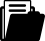Meine Position
i
Ein Tipp vom Eldar Team
Je mehr Sie bestellen, desto grösser Ihr RabattBuch
Buch
Fachbuch
1996

# Nonlinear Integral Equations in Abstract Spaces

ISBN
EAN
978-0-7923-4144-4
9780792341444
Artikel-Nr.
D4VR4P4
Kostenloser Versand
CHF
160.42
Anzahl
1
Maximale
Lieferzeit
13
Arbeitstage
Freitag
15.10.2021Beschreibung
Many problems arising in the physical sciences, engineering, biology and ap plied mathematics lead to mathematical models described by nonlinear integral equations in abstract spaces. The theory of nonlinear integral equations in ab stract spaces is a fast growing field with important applications to a number of areas of analysis as well as other branches of science. This book is devoted to a comprehensive treatment of nonlinear integral equations in abstract spaces. It is the first book that is dedicated to a systematic development of this subject, and it includes the developments during recent years. Chapter 1 introduces some basic results in analysis, which will be used in later chapters. Chapter 2, which is a main portion of this book, deals with nonlin ear integral equations in Banach spaces, including equations of Fredholm type, of Volterra type and equations of Hammerstein type. Some applica equations tions to nonlinear differential equations in Banach spaces are given. We also discuss an integral equation modelling infectious disease as a typical applica tion. In Chapter 3, we investigate the first order and second order nonlinear integro-differential equations in Banach spaces including equations of Volterra type and equations of mixed type. Chapter 4 is devoted to nonlinear impulsive integral equations in Banach spaces and their applications to nonlinear impul sive differential equations in Banach spaces.Stichwörter
Integral equation
banach spaces
development
equation
integral
mathematics
online
presentation
Zielgruppe
Research
Inhaltsverzeichnis
Preface. 1. Preliminaries. 2. Nonlinear Integral Equations in Banach Spaces. 3. Nonlinear Integro-Differential Equations in Banach Spaces. 4. Nonlinear Impulsive Integral Equations in Banach Spaces. References.Herausgeber/-innen
Autor/-in
Publikation
Vereinigte Staaten
30.09.1996Sprache
EnglischFormat
Hardcover
344 Seiten
23 cm
(Höhe)
15 cm
(Breite)
2.1 cm
(Tiefe)
1490 g
(Gewicht)Weitere FormateVersand
Kostenloser Versand: Schweiz & Liechtenstein
Für den Versand nach Deutschland oder Frankreich werden die Versandgebühren der Schweizerischen Post berechnet. Diese werden Ihnen im Warenkorb für Ihre gesamte Bestellung berechnet.Themen
Unterkategorie
Analysis
Zurück
Zum Start